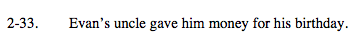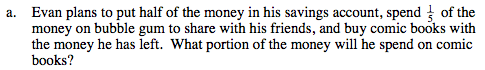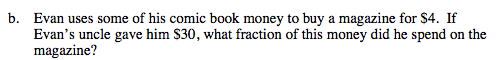### Home > MC2 > Chapter 2 > Lesson 2.1.2 > Problem2-33

2-33.$\text{Evan realized that half is the same as } 50\% \text{ or } \frac{1}{2}$

$\text{What } \% \text{ is } \frac{1}{5} \text{ the same as?}$

With these portions, solve for the remainder to find
what portion Evan still had to spend on comic books.

30% is left over because 100% − 50% − 20% = 30%.Evan spent $4 out of$30. Try writing this as a fraction.